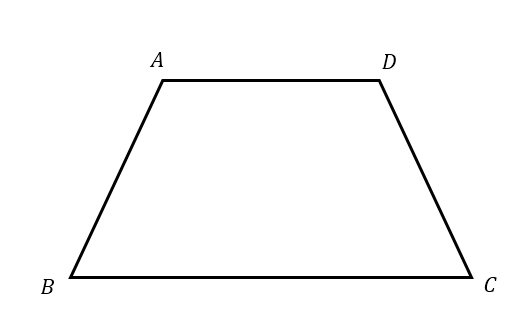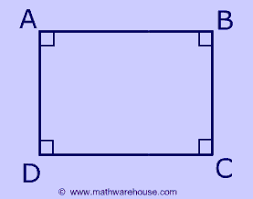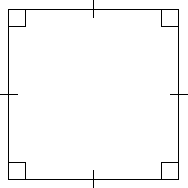Quadrilateral - Trapezium, Parallelogram, Rhombus, Square, Rectangle

According to the Euclidean geometry, quadrilaterals are can be defined as polygons with four sides and four vertices. They are all 2D structures. There are seven types of quadrilaterals.

TRAPEZIUM

A trapezium has no sides which are parallel to each other. An isosceles trapezium has one pair of opposite sides which are parallel to one another and also their base angles are equal to one another.Properties of trapezium

• • The base of the trapezium is parallel to each other. In the above case, AD // BC

• • None of the sides, diagonals and angles are equal to each other.

• Important formulas related to trapezium

• • Area of trapezium = (1/2) h (L+L2)

• • Perimeter of Trapezium = L + L1 + L2 + L3

• PARALLELOGRAM

A parallelogram is a type of quadrilateral in which two pairs of parallel sides are present. In this, the opposite sides are also equal to one another. Parallelograms include rhombus, rhomboid, and rectangles too.Properties of parallelogram

• • The opposite sides of a parallelogram are parallel to one another and are also equal to one another.

• • The angles are also congruent.

• • The adjacent angles are supplementary

• • The diagonals bisect each other and the diagonal divides the parallelogram into two congruent triangles.

• • If one of the angles in the parallelogram is a right angle, then all the other angles are a right angle and the parallelogram becomes a rectangle.

• Important formulas related to parallelograms

• • Area of a parallelogram = L*H

• • Perimeter of parallelogram = 2 (L+B)

• RHOMBUS

A rhombus is a type of quadrilateral in which all the four sides are of equal length. Also, the diagonals are perpendicular to one another and bisect each other too.Properties of rhombus

• • All the sides of a rhombus are equal.

• • The opposite angles are also congruent in a rhombus.

• • The diagonals of a rhombus are perpendicular to each other and also bisect each other.

• • The adjacent angles are supplementary.

• • A rhombus is also a parallelogram whose diagonals are perpendicular to each other.

• Important formulas for rhombus

• • If a and b are the diagonals of the rhombus, then,

• • Area of rhombus = (a* b) / 2

• • The perimeter of a rhombus = 4L

• RHOMBOID

Rhomboid is basically a type of parallelogram in which the adjacent sides are of unequal length and all the angles present are oblique. Rhomboid is parallelogram which is not a rhombus.

RECTANGLE

The rectangle is a parallelogram in which all the four angles present are equivalent to right angles. The diagonals bisect each other and also are equal in length.Properties of rectangle

• • Opposite sides of a rectangle are parallel and congruent.

• • All the angles are a right angle.

• • The diagonals are congruent and also bisect each other.

• • Opposite angles which are formed at the point where the diagonals meet are congruent.

• • A rectangle is a unique type of parallelogram in which all the angles are right.

• Important formulas for rectangle

• • If the breadth is B and the length is L, then,

• • The length of the diagonal of the rectangle is √(L2 + B2)

• • The area of the rectangle is L * B

• • The perimeter of the rectangle is 2 (L+B)

• SQUARE

A square is a quadrilateral in which all the four sides are equal in length and all the angles are equal. All the angles are equal to 90 degrees i.e. they are right angles.

A square is also a parallelogram as the opposite sides are parallel to each other. Also, the diagonals are perpendicular to each other and are also of equal length. A quadrilateral can only be called a square if it satisfies both the properties of rectangle and rhombus.Properties of a square

• • All sides and angles are congruent.

• • Opposite sides are parallel to each other.

• • The diagonals are congruent.

• • The diagonals are perpendicular to and bisect each other.

• • A square is a special type of parallelogram whose angles and sides are equal.

• • Also, a parallelogram becomes a square when the diagonals are equal and right bisectors of each other.

• Important formulas for Squares

• • If ‘L' is the length of the side of a square the length of the diagonal = L √2.

• • Area = L2.

• • Perimeter = 4L

• KITE

Out of the four sides, two adjacent sides are equal. This means that two of the sides are equal and the other two sides are equal. However, they are not equal to each other. None of the sides is parallel. The diagonals are not equal, but they bisect each other at right angles.

Properties of a kite

• The two adjacent sides are equal.
• The diagonals are not equal.
• The diagonals are perpendicular bisectors of each other.
• One diagonal divided the kite into two congruent triangles. This implies that the angles formed by the equal sides are equal.

Important formulas for kite

• • Area = (pq)/2 if p and q are the lengths of the diagonals respectively.